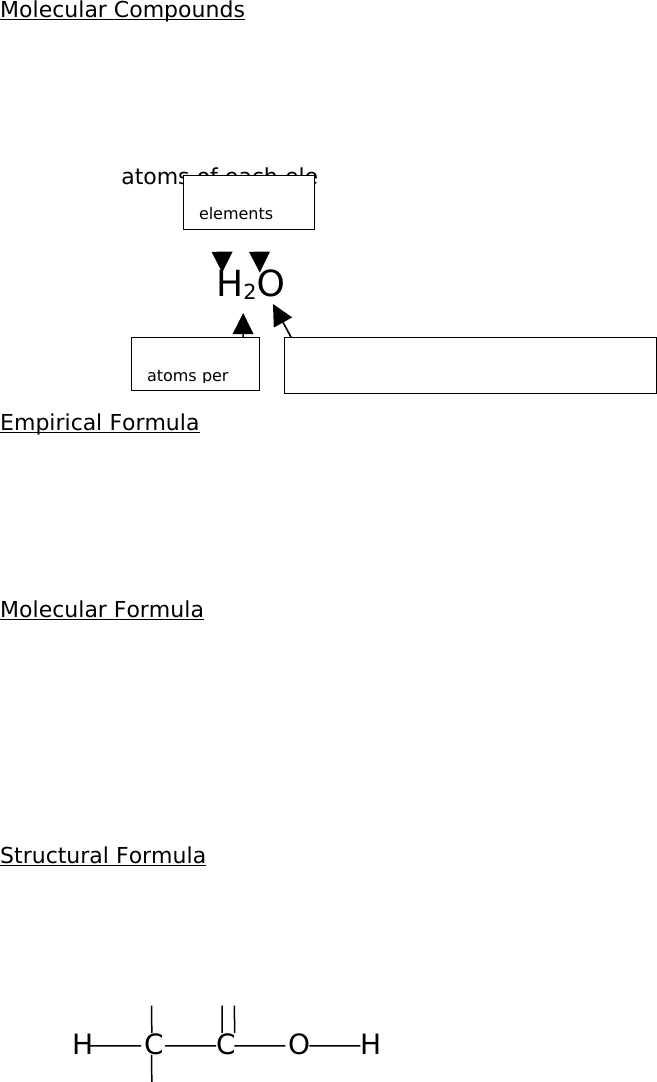# CHEM 499 Lecture Notes - Formula Unit, Hydrogen Iodide, Chlorate

68 views9 pages
Page:
of 9Molecular Compounds
A molecular compound is made up of discrete units called molecules, which
typically consist of a small number of non-metal atoms held together by covalent
bonds. Molecular compounds are represented by chemical formulas, symbolic
representations that, at minimum, indicate: the elements present and the relative
number of atoms of each element.
Example: H2O
Empirical Formula
The empirical formula is the simplest formula for a compound where its subscripts
are reduced to their simplest whole number ratios. Generally, the empirical formula
does not tell one a significant about of information about a given compound. Acetic
acid (C2H4O2) and glucose (C6H12O6) both have the empirical formula CH2O.
Molecular Formula
The molecular formula is based on an actual molecule of a compound. In some
cases, the empirical and molecular formulas are identical, such as formaldehyde
(CH2O). In other cases, the molecular formula is a multiple of the empirical formula.
Both the empirical and molecular formulas provide information on the combining
rations of the atoms in the compounds, however, they show nothing on how the
atoms are attached relative to each other.
Structural Formula
The structural formula shows the order in which atoms are bonded together in a
molecule and by what types of bonds. For example acetic acid:
H O
H C C O H
H
The covalent bonds in the structural formula are represented by lines or dashes and
a double bond is represented by a double dash.
Two H
atoms per
Lack of subscript means 1 atoms of O per
molecule
The 2
elements
Condensed Structural Formula
This formula is a representation of the molecular structure of a compound which is
written in one line and is an alternate way of showing how the atoms of a molecule
are connected. For example with acetic acid: CH3COOH or CH3CO2H.
It can also be used to show how a group of atoms is attached to another atom. For
example, methylpropane (C4H10).
CH3
CH3CH CH3can be written as CH3CH (CH3) CH3 or as CH (CH3)3
CH3
The parentheses indicate that that ligand is connected to the molecule at that given
point.
Line angle Formula
The line angle formula contains lines that represent chemical bonds. For example,
the male hormone molecule, testosterone.
OH
H3C
H3C
O
Ionic Compounds
An ionic compound is made up of positive and negative ions joined together by
electrostatic forces of attraction. The atoms of metallic elements tend to lose one or
more electrons when combining with non-metal atoms. The non-metal atoms, in
return, gains one or more electrons in the process. When the metal loses an
electron during transfer, it becomes a cation (positive ion) and the non-metal atom
becomes an anion (negative ion).
Consider NaCl. An ionic crystal of NaCl shows that one Na+ ion is surrounded by six
Cl- ions. We cannot pick any one of these Cl- ions and exclusively associate it with a
given Na+, however, since the ratio of chloride ions to sodium ions is 1:1, and so a
combination of one sodium ion and one chloride ion as a formula unit. The formula
unit of an ionic compound is the smallest electrically neutral collection of ions. The
ratio of atoms in the formula unit is essentially the same as in the chemical formula.
Since a formula unit is only a fragment of a vast network of ion (crystal), a formula
unit cannot be distinguished as distinct. Therefore, it is incorrect to label a formula
unit as a molecule.
Ions such as Na+, Mg2+ and Cl- are monatomic, meaning that each consists of a
single ionized atom. Alternately, and ion made up of two or more atoms are called
polyatomic ions (e.g. NO3-). (Study polyatomic ions)
The Mole Concept and Chemical Compounds
Formula mass: the mass of a formula unit in atomic mass units. It is appropriate to
use the term formula mass, but for molecular compounds, the formula unit is
actually a molecule, so the term molecular mass is used. The molecular mass is
the mass of a molecule in atomic mass units (amu).
Mole of a Compound
The molar mass is the mass of one mole of compound – one mole of molecules of a
molecular compound and one mole of formula units of an ionic compound.
Problem: An analytical balance can detect a mass of 0.1 mg. What is the total
number of ions present in this minimally detectable quantity of MgCl2?
Solution: Use molar mass to convert from mass to number of moles of MgCl2. Then
use Avogadro constant as a conversion factor to convert moles to number of
formula units (fu). Finally, there are three ions (1 Mg2+ and two Cl-) per formula unit
of magnesium chloride.
? ions =
[0.1mg MgCl2 x (1 g MgCl2 / 1000 mg MgCl2) x (1 mol MgCl2 / 95 g MgCl2) x (6.0 x
10²³ fu MgCl2 / 1 mol MgCl2) x (3 ions / 1 fu MgCl2)]
= 2 x 1018 ions
Problem: The volatile liquid ethyl mercaptan, C2H6S, is one of the most odoriferous
substances known. It is sometimes added to natural gas to make gas leaks
detectable. How many C2H6S molecules are contained in a 1.0 µL sample? (d=0.84
g/mL)
Solution:
? molecules C2H6S =